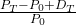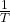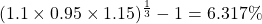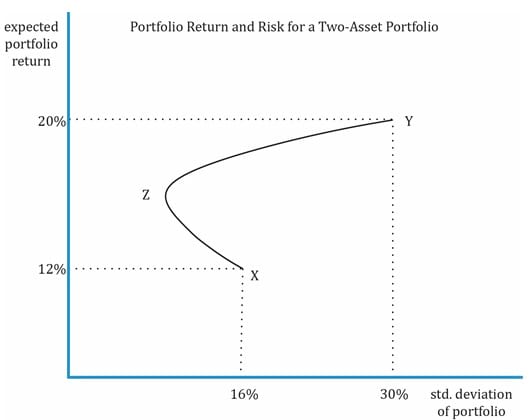IFT Notes for Level I CFA® Program
IFT Notes for Level I CFA® Program

# Part 1

## 1.  Introduction

• Investment characteristics of assets in terms of their return and risk.
• How to determine what assets are appropriate for a portfolio.
• How to construct an indifference curve and use it in the selection of an optimal portfolio using two risky assets.
• How to construct an optimal risky portfolio.

## 2.  Investment Characteristics of Assets

### 2.1.     Return

Return can come in two forms:

• Periodic income through interest payments or dividends.
• Capital gains when the price of the asset you hold increases.

We now look at the various types of return measures and their applicability.

Holding Period Return

Holding period return is the return earned on an asset during the period it was held. It is calculated as a sum of capital gain (price appreciation) and periodic income.

HPR =where:

P– P0 = capital gain component

DT = dividend or income earned during period T

PT = price at end of period

P0 = price at beginning of period

Arithmetic or Mean Return

Arithmetic return is a simple arithmetic average of returns. Assume you have three stocks A, B, C with returns of 10%, 20% and 30% respectively. The collective return from the three stocks is (10 + 20 + 30)/3 = 20%.

Geometric Mean Return

Geometric mean return is the compounded rate of return earned on an investment.

Geometric mean return = [(1 + Ri1) * (1 + Ri2) * …….* (1 + RiT)]– 1

Assume you have a stock A which returns 10%, 20% and 30% in years 1, 2, and 3 respectively.

What is the mean return earned?

Geometric mean return = [(1.1) (1.2) (1.3)]0.333 – 1 = 19.7%

Money-weighted Return or Internal Rate of Return

Money-weighted return is the internal rate of return on money invested that considers the cash inflows and cash outflows, and calculates the return on actual investment.

Money-weighted return is a useful performance measure when the investment manager is responsible for the timing of cash flows. This is often the case for private equity fund managers.

Example

Given the data below, compute the holding period return, arithmetic mean return, geometric mean return, and money-weighted return. Assume no withdrawals except at the end of year 3.

 Year Assets under management at start of year (millions of $) Net return 1 30 10% 2 33 -5% 3 35 15% Solution: Holding period return: Holding period = 3 years Return = 1.1 x 0.95 x 1.15 = 1.20175 = 20.175% Arithmetic mean: Return = (10 – 5 + 15)/3 = 6.67% Geometric mean: Return =Money-weighted return: To calculate the money-weighted return, we must know the net cash flows (cash inflows and outflows) for every year. So, let us draw a table and fill in the values and derive some others (in italics) to get the values for CF0, CF1, CF2, and CF3.  Year 1 Year 2 Year 3 Balance from previous year 0 33.00 31.35 New investment by investor 30.00 0 3.65 Withdrawal by investor 0 0 0 Net balance at start of year 30.00 33.00 35.00 Investment return for year 10% -5% 15% Investment gain (loss) 3.00 (1.65) 5.25 Balance at end of year 33.00 31.35 40.25 Now that we have the cash flows for the three years, let’s use the financial calculator to calculate IRR. CF0 = -30; CF1 = -3.84; CF2 = 0; CF3 = 40.25 IRR = 6.62% Time-Weighted Rate of Return The time-weighted rate of return measures the compound growth rate of$1 initially invested in the portfolio over a stated measurement period.

The time-weighted return can be calculated using the following steps:

1. Break the overall evaluation period into sub-periods based on the dates of cash inflows and outflows.
2. Calculate the holding period return on the portfolio for each sub-period.
3. Link or compound holding period returns to obtain an annual rate of return for the year (the time-weighted rate of return for the year).
4. If the investment is for more than a year, take the geometric mean of the annual returns to obtain the time-weighted rate of return over that measurement period.

Consider the following example:

 Time Outflow Inflow 0 $20.00 to purchase the first share 1$22.50 to purchase the second share $0.50 dividend received on first share$1.00 dividends ($0.50 x 2 shares);$47.00 from selling 2 shares @ $23.50 per share Calculating the TWRR for this example is relatively simple because cash flows only occur at the start/end of every year. We will follow the steps mentioned earlier: Steps 1: Break into evaluation period and value the portfolio at start/end of every period. • Value of the portfolio at the start of Year 1 (t = 0) is$20.00.
• Value of portfolio at the end of Year 1 (t = 1) before the purchase of the new share is 22.50 + 0.50 = $23.00. Note that the dividend of$0.50 on the first share is received at the end of Year 1.
• Value of the portfolio at the start of Year 2 (t = 1) after the purchase of the second share is 22.50 + 22.50 = $45.00. The dividend of$0.50 from the first share is paid out and is not considered as part of the portfolio.
• Value of the portfolio at the end of Year 2 (t = 2) is 23.50 + 23.50 + 0.50 + 0.50 = $48.00. Both shares pay a dividend of$0.50 at the end of the second year.

Step 2: Calculate the holding period return on the portfolio for each sub-period.

• In this question the cash flows are taking place at the start/end of each period. Hence there are no sub-periods. Scenarios involving sub-periods will be covered in the next example.

Step 3: Link or compound holding period returns to obtain an annual rate of return for the year.

• The annual rate of return is based on the portfolio value at the start and end of each period.
• The portfolio value at the start of Year 1 was $20.00 and the value at the end of Year 1 was$23.00. Hence the holding period return was 15.00%.
• The portfolio value at the start of Year 2 was $45.00 and the value at the end of Year 2 was$48.00. Hence the holding period return was 6.67%.

Step 4: If the investment is for more than a year, take the geometric mean of the annual returns to obtain the time-weighted rate of return over that measurement period.

The TWRR is calculated as:  = 0.1077 = 10.77%.

Money-weighted v/s time-weighted returns

• The money-weighted rate of return is impacted by the timing and amount of cash flows.
• The time-weighted rate of return is not impacted by the timing and amount of cash flows.
• The time-weighted return is an appropriate performance measure if the portfolio manager does not control the timing and amount of investment.
• On the other hand, money-weighted return is an appropriate measure if the portfolio manager has control over the timing and amount of investment.

Annualized Return

Annualized return converts the returns for periods that are shorter or longer than a year, to an annualized number for easy comparison.

Annualized Return = (1 + rperiod)c – 1
Where
c = number of periods in year

### 2.2.     Other Major Return Measures and Their Applications

Gross Return

Gross return is the return earned by an asset manager prior to deducting management fees and taxes. It measures the investment skill of a manager.

Net Return

Net return is the return earned by the investor on an investment after all managerial and administrative expenses have been accounted for. This is the measure of return that should matter to an investor.

Assume an investment manager generates $120 for every$100, and charges a 2% fee for management and administrative expenses. The gross return, in this case, is 20% and the net return is 18%.

Pre-tax and After-tax Nominal Return

The returns we saw till now were pre-tax nominal returns, i.e., before deducting any taxes or any adjustments for inflation. This is the default, unless otherwise stated.

After-tax nominal return is the return after accounting for taxes. The actual return an investor earns should consider the tax implications as well.

In the example that we saw above for gross and net return, 18% was the pre-tax nominal return. If the tax rate for the investor is 33.33%, then the after-tax nominal return will be 18(1 – 0.3333) = 12.0006%.

Real Return

Real return is the return after deducting taxes and inflation.

(1 + r) = (1 + rreal) (1 + π)

where:

rreal = real rate

π = rate of inflation

r = nominal rate

In the previous example, the after-tax nominal return was 12%. Assume the inflation rate for the period is 10%. What is the real rate of return?

Using the above formula, (1 + 0.12) = (1 + r) (1 + 0.1). Solving for r, we get 1.818%.

Instructor’s tip: If the answer choices are close to each other, use this formula to determine the correct answer. Else, you may use an approximation to solve for r quickly as nominal rate = real rate + inflation.

Leveraged Return

In cases, where an investor borrows money to invest in assets like bonds or real estate, the leveraged return is the return earned by the investor on his money after accounting for interest paid on borrowed money.

Portfolio Expected Return and Variance

For a two-asset portfolio, the expected return and variance can be computed as:

E(RP) = w1R1 + w2R2

𝜎p2 = w12 𝜎12 + w22 𝜎22 + 2 w1 w2 𝜎1 𝜎2 ρ

Example

My portfolio consists of two stocks X and Y. X represents 60% of the portfolio and Y the remaining 40%. X has an expected return of 12% and a standard deviation of 16%. Y has an expected return of 20% and a standard deviation of 30%. The correlation is 0.5. What is the expected return and risk of my portfolio? How does the return/risk change when the weights of X and Y change?

Solution:

We can calculate the portfolio variance:

𝜎p2 = (0.6)2 (0.16)2 + (0.4)2 (0.3)2 + 2 (0.6) (0.4) (0.16) (0.3) (0.5) = 0.0351

𝜎p = 0.1874 = 18.74%

To understand how return/risk change when the weights of X and Y change, we will calculate the risk and return for different weights and plot a curve as shown in the graph below.

• Point X represents 100% of the portfolio invested in stock X with return of 12% and standard deviation of 16%.
• Point Y represents 100% of the portfolio invested in stock Y with expected return of 20% and a standard deviation of 30%.
• The curve between X and Z (to the left of X) represents the region where amount invested in stock X is decreased while the weight of Y is increased. This region is where the benefit of diversification is seen, i.e., the expected return increases while risk goes lower.
• Beyond the Point Z, when the weight of Y is increased till the point Y where 100% is invested in Y, there is no diversification benefit. For any point between Z and Y, the risk and return increase.

The graph below plots portfolio risk and return for a two-asset portfolio and shows the impact of correlation of assets on portfolio risk. As you can see, there is no risk-return trade-off when 100% is invested either in X or Y.